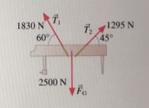# Problem: The two ropes seen In the figure are used to lower a 255 kg piano 6.00 m from a second-story window to the ground.How much work is done by each of the three forces ?

###### FREE Expert Solution

Work done:

$\overline{){\mathbf{W}}{\mathbf{=}}{\mathbf{F}}{\mathbf{·}}{\mathbf{d}}}$

The horizontal components of the forces sum up to zero.

Only the vertical components of the force do work.

88% (311 ratings)###### Problem Details

The two ropes seen In the figure are used to lower a 255 kg piano 6.00 m from a second-story window to the ground.How much work is done by each of the three forces ?